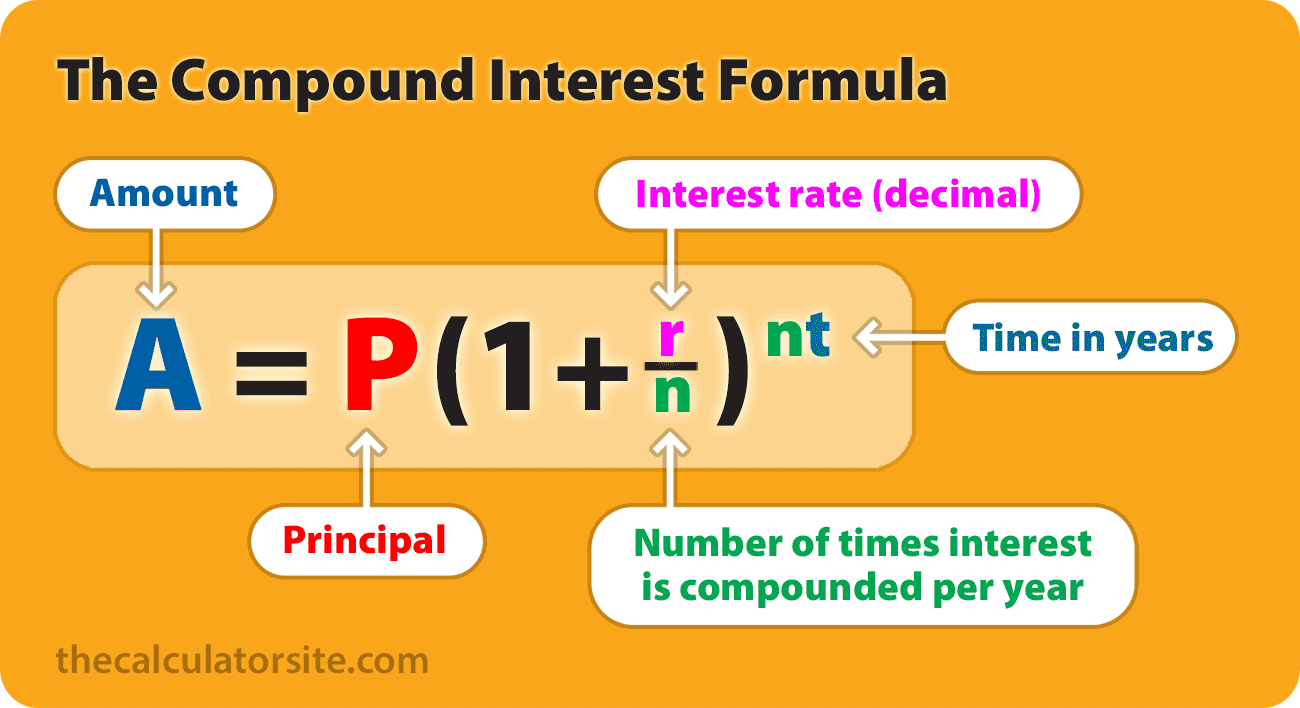# Compound Interest Calculator

## How to calculate your interest

To begin your calculation, enter your starting amount along with the annual interest rate and the start date (assuming it isn’t today). Then, enter a number of years, months or days that the calculation is to run. Once you click the ‘calculate’ button, the simple interest calculator will show you:

• The interest accrued
• The split of final value## Simple interest calculator formula

Our calculator uses the following formula:

## FV = P(1 + rt)

Where:

• FV = Final value (principal + interest)
• P = Principal (starting amount)
• r = Annual interest rate (as decimal)
• t = time period (in years)

Should you wish to work out the total interest only, simply calculate FV – P.

## Example interest calculation

If an amount of \$5,000 is invested at a yearly interest rate of 5%, with no compounding (simple interest), its value after 3 years can be calculated as follows…

P = 5000.
r = 5/100 = 0.05 (decimal).
t = 3.

Plugging those figures into the formula gives the following:

FV = 5000 * (1 + (0.05 * 3))

FV = 5000 * 1.15 = 5750

The balance after 3 years is therefore 5,750.